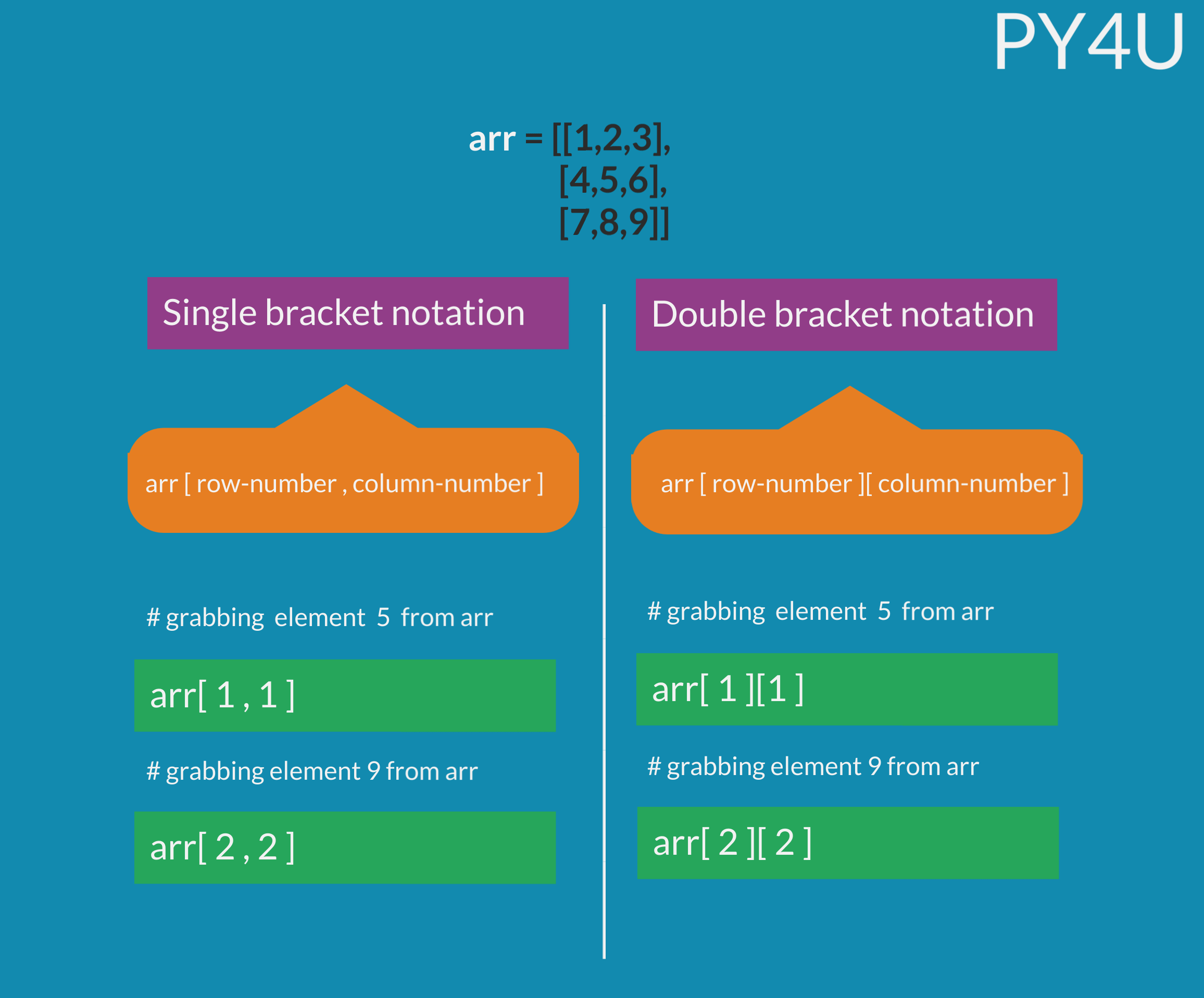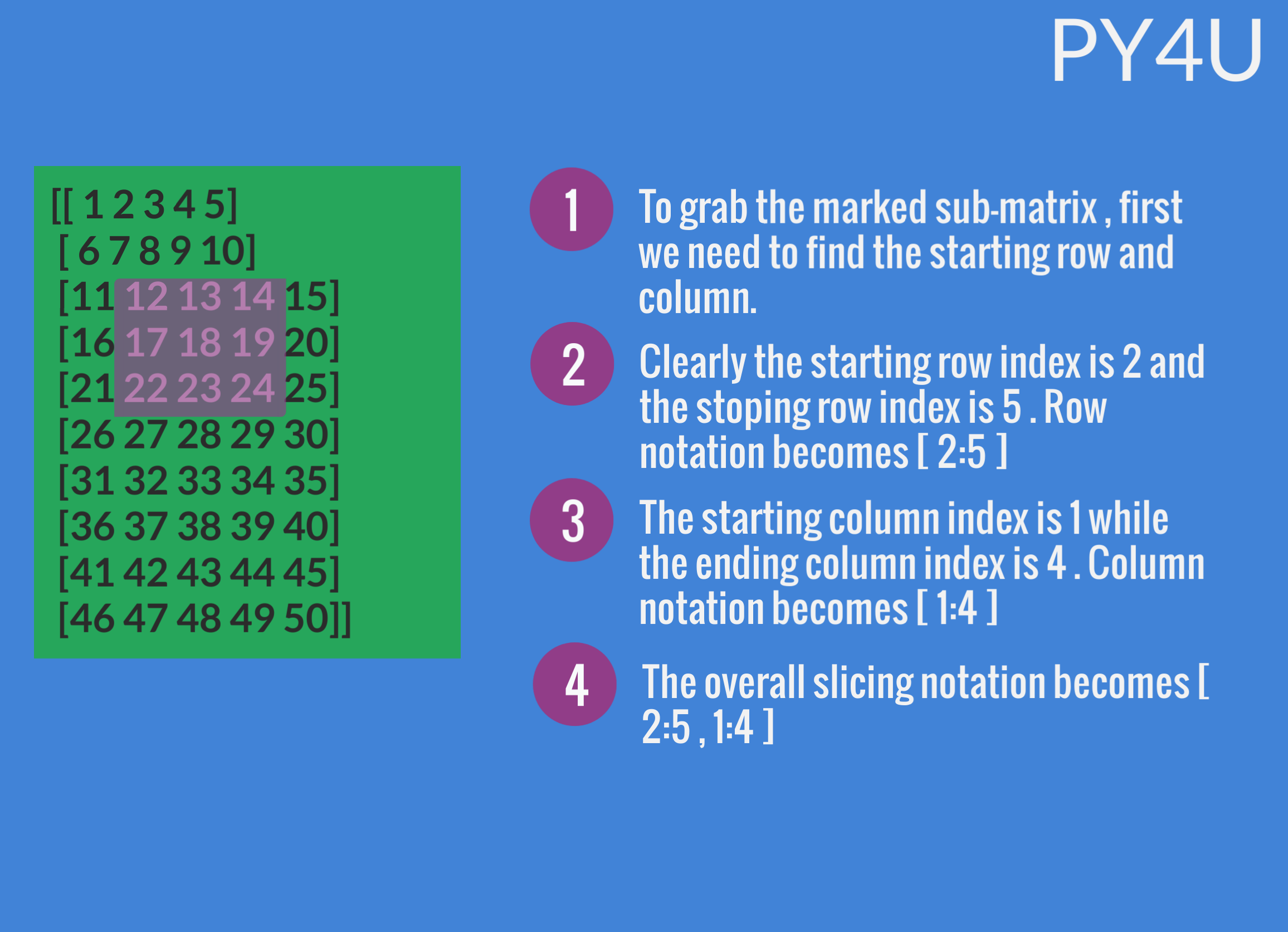Indexing In Arrays

Indexing and slicing in arrays is very much similar to that of an list. Let us first learn indexing in an 1-D array.

In the code given below , i have a 1-D array and i'll be grabing single and multiple elements from it.

import numpy as np
arr = np.arange(1,51)

#let us grab number 25
print(arr)

#let us grab number 40
print(arr)

#let us grab all the numbers from 25 till the end
print(arr[24:])

#let us grab all the odd  numbers from start till 40
print(arr[:39:2])
Output :
25
40
[25 26 27 28 29 30 31 32 33 34 35 36 37 38 39 40 41 42 43 44 45 46 47 48
49 50]
[ 1  3  5  7  9 11 13 15 17 19 21 23 25 27 29 31 33 35 37 39]

Let us learn indexing in a 2-D array. There are two ways in which indexing and slicing can be done:

• Single square bracket notation
• Double square bracket notation

In the upcoming examples , we'll be using both notations to grab elements so that you can find the one that suits you. Before that let us quickly look at the basic syntax difference between the two.Let us look at a few examples where we perform indexing and slicing on 2-D arrays.

import numpy as np
my_arr =np.arange(1,51).reshape(10,5)

#let us grab the whole 3rd row
print(my_arr[0:])
print()

#let us grab 3 x 3 matrix from 1st to 3rd row and 3rd to 5th column
print(my_arr[0:3,2:])
print()

#let us grab the whole 5th column
print(my_arr[0:,4])
Output :
[11 12 13 14 15]

[[ 3  4  5]
[ 8  9 10]
[13 14 15]]

[ 5 10 15 20 25 30 35 40 45 50]

Having trouble grabbing a sub-matrix from a matrix ?? don't worry. In the infographic below , i will be explaining it in steps.Conditional selection

Conditional selection allows arrays to filter out content based on certain conditions.

import numpy as np
my_arr = np.array([1,2,3,4,5,6,7])
print(my_arr[my_arr >= 2])
print(my_arr[my_arr >= 5])
Output :
[2 3 4 5 6 7]
[5 6 7]

Popular Tutorials